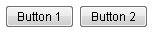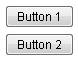# Box

• Demonstration: Box
• Java API: Box
• JavaScript API: Box
• Style Guide: Box

# Employment/Purpose

The box model of XUL is used to divide a portion of the display into a series of boxes. Components inside a box will orient themselves horizontally or vertically. By combining a series of boxes and separators, you can control the layout of the visual presentation.

A box can lay out its children in one of two orientations, either horizontally or vertically. A horizontal box lines up its components horizontally and a vertical box orients its components vertically. You can think of a box as one row or one column from an HTML table.

A box is the generic component that can be used for horizontal and vertical layouts. However, it is generally more convenient by the use of hbox and vbox directly.

Notice that hbox and vbox are designed to provide more sophisticated layout, such as splitter, alignment and packing. If you need only the layout feature, it is suggest to use Hlayout and Vlayout instead, since the performance is much better (due to the use of HTML DIV instead of TABLE).

# Example

``` <zk>
<box orient="vertical">
<button label="Button 1"/>
<button label="Button 2"/>
</box>
<box  orient="horizontal">
<button label="Button 3"/>
<button label="Button 4"/>
</box>
</zk>```

# Properties

## Spacing

You can control the spacing among children of the box control. For example, the following example puts 5em at both the upper margin and the lower margin. Notice: the total space between two input fields is 10em.

```<vbox spacing="5em">
<textbox/>
<datebox/>
</vbox>```

Another example illustrated an interesting layout by the use of zero spacing.

```<window title="Box Layout Demo" border="normal">
<hbox spacing="0">
<window border="normal">0</window>
<vbox spacing="0">
<hbox spacing="0">
<window border="normal">1</window>
<window border="normal">2</window>
<vbox spacing="0">
<window border="normal">3</window>
<window border="normal">4</window>
</vbox>
</hbox>
<hbox spacing="0">
<vbox spacing="0">
<window border="normal">5</window>
<window border="normal">6</window>
</vbox>
<window border="normal">7</window>
<window border="normal">8</window>
<window border="normal">9</window>
</hbox>
</vbox>
</hbox>
</window>```

## Heights and Widths

You can control the width for each cell inside a hbox with widths attribute as follows (don't specify on each cell):

```    <hbox width="100%" height="100px" widths="10%,20%,30%,40%" >
<label value="10%"/>
<label value="20%"/>
<label value="30%"/>
<label value="40%"/>
</hbox>
<vbox width="100%" height="500px" heights="10%,20%,30%,40%" pack="stretch">
<label value="10%"/>
<label value="20%"/>
<label value="30%"/>
<label value="40%"/>
</vbox>```

The value is a comma-separated list of widths. If any value is missed, no width is generated for the corresponding cell and the real width is up to the browser.

Similarly, you can specify the height of each cell inside a vbox using the heights attribute. These two properties are the same since the orientation of a box can be horizontal or vertical depending on the orient property.

## Align and Pack

```<zk xmlns:n="http://www.zkoss.org/2005/zk/native">
<zscript><![CDATA[
Map map = new LinkedHashMap();
String[] packs = new String[]{"", "start", "center", "end"};
String[] aligns = new String[]{"", "stretch", "start", "center", "end"};

for (int i = 0; i < aligns.length; i++) {
String align = aligns[i];
List list = new ArrayList();
for (int j = 0; j < packs.length; j++) {
}
map.put(align, list);
}

]]></zscript>
<tablelayout columns="6">
<tablechildren >
<panel border="normal" height="150px" width="100px"
forEach='"pack / align", "", "start", "center", "end"'>
<panelchildren>
<n:h3>\${each}</n:h3>
</panelchildren>
</panel>
</tablechildren>
<tablechildren forEach="\${map}">
<variables key="\${each.key}"/>
<panel border="normal" height="150px" width="100px">
<panelchildren>
<n:h3>\${key}</n:h3>
</panelchildren>
</panel>
<panel border="normal" height="150px" width="100px" forEach="\${each.value}" >
<panelchildren>
<box align="\${key}" pack="\${each}" height="90%" width="100%">
<button label="1" />
<button label="2" />
<button label="3" />
</box>
</panelchildren>
</panel>
</tablechildren>
</tablelayout>
</zk>```
```[Since 5.0.0]
```

# Cell Component

In ZK5, we have introduced a new component named Cell which can be embedded into a Grid or Box (Hbox and Vbox) to fully control the layout and the style. You can now use the rowspan or the colspan property to layout your Grid, for example a content cell can now cross over multiple rows. The code below demonstrates how to do this:

```<box>
<cell sclass="years">
...
</cell>
</box>```
```[Since 5.0.0]
```

# Limitation

Box component is consisted by Table element. Therefore, when put Input element like Textbox, Combobox inside Box component, specify width and height to Box component will be ignored when browser try to render table element.

For example,

```<hbox height="200px" width="200px" style="border: 1px solid red">
<textbox hflex="1" value="1" />
<textbox hflex="1" value="1" />
</hbox>```

You will see the Box width exceed 200px. Also check the sample with pure HTML in jsfiddle.

Name
Event Type
None None

# Supported Molds

Available molds of a component are defined in lang.xml embedded in zul.jar.

Name
Snapshot
horizontalvertical```*ALL
```

# Use Cases

Version Description Example Location

# Version History

Last Update : 2019/5/7

Version Date Content
5.0.4 August, 2010 Add a sizedByContent method for splitter to resize smoothly